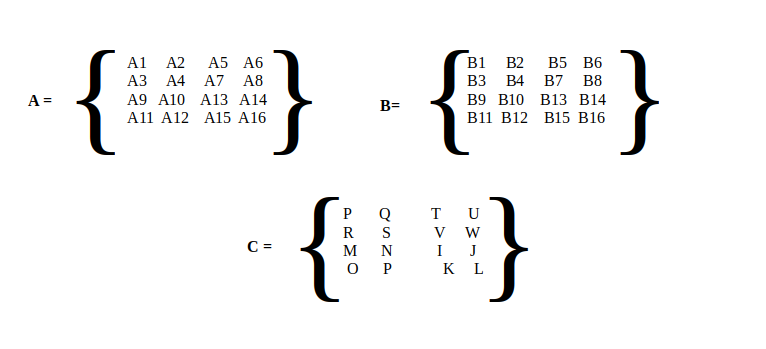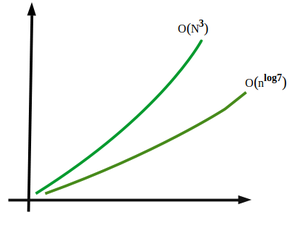# Implementing Strassen’s Algorithm in Java

• Difficulty Level : Hard
• Last Updated : 13 Aug, 2021

Strassen’s algorithm is used for the multiplication of Square Matrices that is the order of matrices should be  (N x N).  Strassen’s Algorithm is based on the divide and conquer technique. In simpler terms, it is used for matrix multiplication. Strassen’s method of matrix multiplication is a typical divide and conquer algorithm. However, let’s get again on what’s behind the divide and conquer approach and implement it considering an illustration as follows For example: Let A and B are two matrices then the resultant matrix C such that

Matrix C = Matrix A * Matrix B

Consider for now the mathematical computation of matrices is that it can be concluded out why the implementation for the Strassen matrices comes out into play. Suppose two matrices are operated to be multiplied then the approach would have been

1. Take input of two matrices.
2. Check the compatibility of matrix multiplication which holds true only and only if the number of rows of the first matrix equals the number of columns of the second matrix.
3. Multiply the matrix and assign multiplication of two matrices to another matrix known as the resultant matrix.
4. Print the resultant matrix.

In the above approach, two assumptions are drawn which show why Strassen’s algorithm need arises into play

• Firstly, the time complexity of the algorithm is O(n3) which is too high.
• Secondly, the multiplication of more than two matrices will not only increase the confusion and complexity of the program but also increase the time complexity accordingly.

Purpose:

Volker Strassen’s is a name who published his algorithm to prove that the time complexity O(n3) of general matrix multiplication wasn’t optimal. So it was published Strassen’s matrix chain multiplication and reduced the time complexity. This algorithm is faster than standard matrix multiplication and is useful when numerous large matrices multiplication is computed in the daily world.

Strassen’s Algorithm for Matrix Multiplication

Step 1: Take three matrices to suppose A, B, C where C is the resultant matrix and  A and B are Matrix which is to be multiplied using Strassen’s Method.Step 2: Divide A, B, C Matrix into four (n/2)×(n/2) matrices and take the first part of each as shown below

Step 3: Use the below formulas for solving part 1 of the matrix

```M1:=(A1+A3)×(B1+B2)
M2:=(A2+A4)×(B3+B4)
M3:=(A1−A4)×(B1+A4)
M4:=A1×(B2−B4)
M5:=(A3+A4)×(B1)
M6:=(A1+A2)×(B4)
M7:=A4×(B3−B1)

Then,

P:=M2+M3−M6−M7
Q:=M4+M6
R:=M5+M7
S:=M1−M3−M4−M5```

Step 4: After Solving the first part, compute the second, third, and fourth, and as well as final output, a multiplied matrix is generated as a result as shown in the above image.

Step 5: Print the resultant matrix.

Implementation:

Example

## Java

 `// Java Program to Implement Strassen Algorithm` `// Class Strassen matrix multiplication``public` `class` `GFG {` `    ``// Method 1``    ``// Function to multiply matrices``    ``public` `int``[][] multiply(``int``[][] A, ``int``[][] B)``    ``{``        ``// Order of matrix``        ``int` `n = A.length;` `        ``// Creating a 2D square matrix with size n``        ``// n is input from the user``        ``int``[][] R = ``new` `int``[n][n];` `        ``// Base case``        ``// If there is only single element``        ``if` `(n == ``1``)` `            ``// Returning the simple multiplication of``            ``// two elements in matrices``            ``R[``0``][``0``] = A[``0``][``0``] * B[``0``][``0``];` `        ``// Matrix``        ``else` `{``            ``// Step 1: Dividing Matrix into parts``            ``// by storing sub-parts to variables``            ``int``[][] A11 = ``new` `int``[n / ``2``][n / ``2``];``            ``int``[][] A12 = ``new` `int``[n / ``2``][n / ``2``];``            ``int``[][] A21 = ``new` `int``[n / ``2``][n / ``2``];``            ``int``[][] A22 = ``new` `int``[n / ``2``][n / ``2``];``            ``int``[][] B11 = ``new` `int``[n / ``2``][n / ``2``];``            ``int``[][] B12 = ``new` `int``[n / ``2``][n / ``2``];``            ``int``[][] B21 = ``new` `int``[n / ``2``][n / ``2``];``            ``int``[][] B22 = ``new` `int``[n / ``2``][n / ``2``];` `            ``// Step 2: Dividing matrix A into 4 halves``            ``split(A, A11, ``0``, ``0``);``            ``split(A, A12, ``0``, n / ``2``);``            ``split(A, A21, n / ``2``, ``0``);``            ``split(A, A22, n / ``2``, n / ``2``);` `            ``// Step 2: Dividing matrix B into 4 halves``            ``split(B, B11, ``0``, ``0``);``            ``split(B, B12, ``0``, n / ``2``);``            ``split(B, B21, n / ``2``, ``0``);``            ``split(B, B22, n / ``2``, n / ``2``);` `            ``// Using Formulas as described in algorithm` `            ``// M1:=(A1+A3)×(B1+B2)``            ``int``[][] M1``                ``= multiply(add(A11, A22), add(B11, B22));``          ` `            ``// M2:=(A2+A4)×(B3+B4)``            ``int``[][] M2 = multiply(add(A21, A22), B11);``          ` `            ``// M3:=(A1−A4)×(B1+A4)``            ``int``[][] M3 = multiply(A11, sub(B12, B22));``          ` `            ``// M4:=A1×(B2−B4)``            ``int``[][] M4 = multiply(A22, sub(B21, B11));``          ` `            ``// M5:=(A3+A4)×(B1)``            ``int``[][] M5 = multiply(add(A11, A12), B22);``          ` `            ``// M6:=(A1+A2)×(B4)``            ``int``[][] M6``                ``= multiply(sub(A21, A11), add(B11, B12));``          ` `            ``// M7:=A4×(B3−B1)``            ``int``[][] M7``                ``= multiply(sub(A12, A22), add(B21, B22));` `            ``// P:=M2+M3−M6−M7``            ``int``[][] C11 = add(sub(add(M1, M4), M5), M7);``          ` `            ``// Q:=M4+M6``            ``int``[][] C12 = add(M3, M5);``          ` `            ``// R:=M5+M7``            ``int``[][] C21 = add(M2, M4);``          ` `            ``// S:=M1−M3−M4−M5``            ``int``[][] C22 = add(sub(add(M1, M3), M2), M6);` `            ``// Step 3: Join 4 halves into one result matrix``            ``join(C11, R, ``0``, ``0``);``            ``join(C12, R, ``0``, n / ``2``);``            ``join(C21, R, n / ``2``, ``0``);``            ``join(C22, R, n / ``2``, n / ``2``);``        ``}` `        ``// Step 4: Return result``        ``return` `R;``    ``}` `    ``// Method 2``    ``// Function to subtract two matrices``    ``public` `int``[][] sub(``int``[][] A, ``int``[][] B)``    ``{``        ``//``        ``int` `n = A.length;` `        ``//``        ``int``[][] C = ``new` `int``[n][n];` `        ``// Iterating over elements of 2D matrix``        ``// using nested for loops` `        ``// Outer loop for rows``        ``for` `(``int` `i = ``0``; i < n; i++)` `            ``// Inner loop for columns``            ``for` `(``int` `j = ``0``; j < n; j++)` `                ``// Subtracting corresponding elements``                ``// from matrices``                ``C[i][j] = A[i][j] - B[i][j];` `        ``// Returning the resultant matrix``        ``return` `C;``    ``}` `    ``// Method 3``    ``// Function to add two matrices``    ``public` `int``[][] add(``int``[][] A, ``int``[][] B)``    ``{` `        ``//``        ``int` `n = A.length;` `        ``// Creating a 2D square matrix``        ``int``[][] C = ``new` `int``[n][n];` `        ``// Iterating over elements of 2D matrix``        ``// using nested for loops` `        ``// Outer loop for rows``        ``for` `(``int` `i = ``0``; i < n; i++)` `            ``// Inner loop for columns``            ``for` `(``int` `j = ``0``; j < n; j++)` `                ``// Adding corresponding elements``                ``// of matrices``                ``C[i][j] = A[i][j] + B[i][j];` `        ``// Returning the resultant matrix``        ``return` `C;``    ``}` `    ``// Method 4``    ``// Function to split parent matrix``    ``// into child matrices``    ``public` `void` `split(``int``[][] P, ``int``[][] C, ``int` `iB, ``int` `jB)``    ``{``        ``// Iterating over elements of 2D matrix``        ``// using nested for loops` `        ``// Outer loop for rows``        ``for` `(``int` `i1 = ``0``, i2 = iB; i1 < C.length; i1++, i2++)` `            ``// Inner loop for columns``            ``for` `(``int` `j1 = ``0``, j2 = jB; j1 < C.length;``                 ``j1++, j2++)` `                ``C[i1][j1] = P[i2][j2];``    ``}` `    ``// Method 5``    ``// Function to join child matrices``    ``// into (to) parent matrix``    ``public` `void` `join(``int``[][] C, ``int``[][] P, ``int` `iB, ``int` `jB)` `    ``{``        ``// Iterating over elements of 2D matrix``        ``// using nested for loops` `        ``// Outer loop for rows``        ``for` `(``int` `i1 = ``0``, i2 = iB; i1 < C.length; i1++, i2++)` `            ``// Inner loop for columns``            ``for` `(``int` `j1 = ``0``, j2 = jB; j1 < C.length;``                 ``j1++, j2++)` `                ``P[i2][j2] = C[i1][j1];``    ``}` `    ``// Method 5``    ``// Main driver method``    ``public` `static` `void` `main(String[] args)``    ``{``        ``// Display message``        ``System.out.println(``            ``"Strassen Multiplication Algorithm Implementation For Matrix Multiplication :\n"``);` `        ``// Create an object of Strassen class``        ``// in he main function``        ``GFG s = ``new` `GFG();` `        ``// Size of matrix``        ``// Considering size as 4 in order to illustrate``        ``int` `N = ``4``;` `        ``// Matrix A``        ``// Custom input to matrix``        ``int``[][] A = { { ``1``, ``2``, ``3``, ``4` `},``                      ``{ ``4``, ``3``, ``0``, ``1` `},``                      ``{ ``5``, ``6``, ``1``, ``1` `},``                      ``{ ``0``, ``2``, ``5``, ``6` `} };` `        ``// Matrix B``        ``// Custom input to matrix``        ``int``[][] B = { { ``1``, ``0``, ``5``, ``1` `},``                      ``{ ``1``, ``2``, ``0``, ``2` `},``                      ``{ ``0``, ``3``, ``2``, ``3` `},``                      ``{ ``1``, ``2``, ``1``, ``2` `} };` `        ``// Matrix C computations` `        ``// Matrix C calling method to get Result``        ``int``[][] C = s.multiply(A, B);` `        ``// Display message``        ``System.out.println(``            ``"\nProduct of matrices A and  B : "``);` `        ``// Iterating over elements of 2D matrix``        ``// using nested for loops` `        ``// Outer loop for rows``        ``for` `(``int` `i = ``0``; i < N; i++) {``            ``// Inner loop for columns``            ``for` `(``int` `j = ``0``; j < N; j++)` `                ``// Printing elements of resultant matrix``                ``// with whitespaces in between``                ``System.out.print(C[i][j] + ``" "``);` `            ``// New line once the all elements``            ``// are printed for specific row``            ``System.out.println();``        ``}``    ``}``}`
Output
```Strassen Multiplication Algorithm Implementation For Matrix Multiplication :

Product of matrices A and  B :
7 21 15 22
8 8 21 12
12 17 28 22
8 31 16 31 ```

Time Complexity Of Strassen’s MethodBy Analysis the time complexity Function can be written as:

`T(N) = 7T(N/2) +  O(N2)`

By Solving this using  Master Theorem we get :

`T(n)=O(nlog7)`

Thus time Complexity Of Strassen’s  Algorithm for matrix multiplication is derived as:

`O(nlog7) = O (n2.81)`

O(n3)  Vs O(n2.81)

My Personal Notes arrow_drop_up